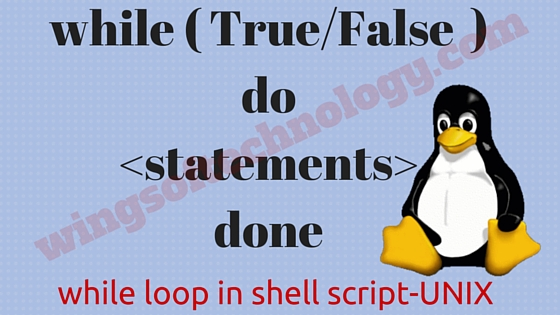# What is while loop in shell script?The shell while loop statement continually executes a block of statements while a particular condition is true.

Syntax for while loop in shell script is:

```while (expression or command)

do

Statement(s) will execute if expression is true

done```

After evaluating shell command, if the resulting value is true then the given statement(s) inside the while are executed.

If command result is false then no statement would be not executed and program come out from the loop and would jump to the next line after done statement.

### How do I use while as infinite loops?

Infinite for while can be created with empty expressions, such as:

#!/bin/bash
while :
do
echo “infinite loop [ hit CTRL+C to stop]”
done

You need to press “Ctrl+C” to stop the loop.

Example for while loop:

Below  is a simple example that uses the while loop to display the numbers zero to ten

#!/bin/bash
var=1
while [ \$var -lt 11 ]
do
echo \$var
a=`expr \$var + 1`
done

Above while loop code will output the following result.

[wot@unix ~] \$ sh while_loop_unix.sh
1
2
3
4
5
6
7
8
9
10
[wot@unix ~]

Each time this loop executes, the variable var is checked to verify whether \$var has a value that is less than 11. If the value of \$var is less than 11, this test condition has an exit status of 0.

In this case, the current value of var is displayed and then var is incremented by 1. This will be done until the shell command is false.

comments